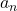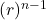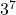## 18,54, 162, … Find the 8th term.

Question

18,54, 162, …
Find the 8th term.

in progress 0
2 months 2021-07-24T15:05:59+00:00 1 Answers 2 views 0

a₈ = 39366

Step-by-step explanation:

The terms have a common ratio between consecutive terms

r = 54 ÷ 18 = 162 ÷ 54 = 3

This indicates the sequence is geometric with nth term= a₁where a₁ is the first term and r the common ratio

Here a₁ = 18 and r = 3 , then

a₈ = 18 ×= 18 × 2187 = 39366